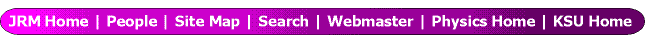Hyperspherical approach to three- and four-electron atomic systems

Toru Morishita
Department of Applied Physics and Chemistry, The University of Electro-Communications,
1-5-1 Chofu-ga-oka, Chofu-shi, Tokyo 182-8585, Japan

C. D. Lin
Department of Physics, Kansas State University, Manhattan, KS 66506, USA

Hyperspherical coordinates were first introduced by Fano and his coworkers [1,2] to understand the basic properties of doubly excited states of helium atoms. In the past several decades, this approach has been improved and extended to a broad range of few-body atomic and molecular systems. In this work, we present our recent progress on the understanding of electron correlations mainly in triply excited states of atoms. We also present a new development of the hyperspherical approach to a four-electron atomic system.

In the hyperspherical method, 3N dimensional configuration space of an N-electron atom with the nucleus at the center is parametrized by a hyperradius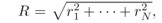characterizing the mean square of the size of the system, and by (3N-1) bounded hyperangles Ω, describing the relative positions of the electrons. In hyperspherical coordinates, the Schrödinger equation can be written as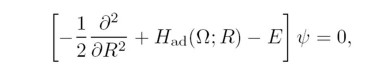where Had(Ω;R) is the adiabatic Hamiltonian which depends parametrically on R. Within the adiabatic approximation introduced by Macek , the total wavefunction for the n-th state in channel μ can be written as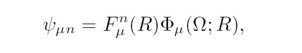where Fμn(R) is the hyperradial function which measures the size of the state; Φμ(Ω;R) is the hyperspherical adiabatic channel function, which contains all the information about electron correlations for states within channel µ. The channel function Φμ(Ω;R) and its associated adiabatic potential Uμ(R) are obtained by solving the adiabatic eigenvalue problem at each R,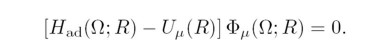We solved this eigenvalue problem for three-electron atomic systems for each 2S+1Lπ symmetry , and for a four-electron atomic system.

As an example of three-electron atoms, we show the adiabatic potential curves for Li(2Se) states in Fig. 1. At large R, each curve approaches the two electron Li+ states: The potentials can be classified into three groups by their asymptotic limits. The first group consists of a single curve - the lowest curve, labeled I in Fig. 1. This curve approaches the Li+(1s2 1Se) state as R → ∞. This curve supports the ground state and the 1s2ns 2Se (n ≥ 2) singly excited states. The second group, labeled II in Fig. 1, consists of potential curves that approach the 1snl (n ≥ 2) singly excited states of Li+ at large R. These curves support doubly excited states. The third group, labeled III, consists of potential curves that approach the nln'l' (n, n' ≥ 2) doubly excited states of Li+ at large R. These curves support triply excited states. Clearly, the avoided crossings among the di.erent groups are very sharp. Thus, the hyperspherical adiabatic channels can be used to separate singly, doubly, and triply excited states.

To identify the features that characterize the adiabatic channels among the triply excited states, we examined the channel functions, Φs. We classify triply excited states of Li in the 2l2l'2l" and the 2l2l'3l" manifolds by visualizing the channel functions in the body-fixed frame. We found that angular correlations play an important role in characterizing the 2l2l'2l" intrashell states. By examining the wavefunctions of the three electrons at the same distance from the nucleus, we found that these intrashell states are distinguished by their distributions in the three relative angles (See Fig. 2 for the definition of the angles). By examining the wave functions in the body-fixed frame, we identi.ed three basic modes of the three relative angles. In Fig. 3, we plotted the equidensity surfaces of internal wavefunctions for the 2l2l'2l" intrashell states. These surfaces can be grouped clearly into three types. In fact, the major distinction being that in group A, the three electrons form a coplanar e- quilateral triangle; in group B they form an equilateral triangle but not coplanar, and in group C the three electrons are coplanar but not allowed to make an equilateral triangle. The forbidden region for the latter two groups originates from the quantum symmetry in that a state with well-defined quantum numbers L, S, and π would incur nodal surfaces in a multidimensional wavefunction.

We also examined the internal wavefunctions for the 2l2l'3l" intershell triply excited states of Li. For these states, radial correlations as well as angular correlations play an important role in the classification. A new quantum number was introduced to describe the symmetric and antisymmetric stretches between the two inner electrons with the third one.

The next major step is to understand the four-electron atomic systems, where one can expect much richer electron correlation effects. We implemented a pilot calculation for Be within the s4 configurations, and resulting hyperspherical adiabatic potential curves for the 1Se symmetry are shown in Fig. 4. The general appearance of the adiabatic potentials does not di.er markedly from those of three-electron atoms such as Li. At large R, each curve converges to the three-electron Be+ states. The groups labeled I, II, and III consist of potential curves that support singly, doubly, and triply excited states of Be, respectively, and they are similar to the three-electron systems as shown in Fig. 1. In addition to these three groups, we can clearly observe the fourth group, labeled IV. The curves in the fourth group converge to the triply excited states of Be+ and they support quadruply excited states of Be. We note that the avoided crossings among the different groups are very sharp. This suggests that quadruply excited states of Be are rather stable against autoionization to singly, doubly, and triply ionized states of Be. Our next goal is to examine the hyperspherical potentials including the effects from higher angular momentum states and to classify the quadruply excited states.

Figures: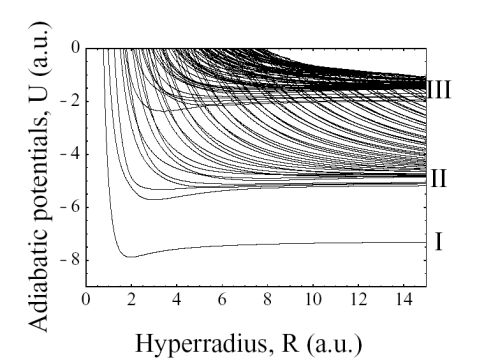Figure 1: Hyperspherical adiabatic potentials for the 2Se symmetry of Li.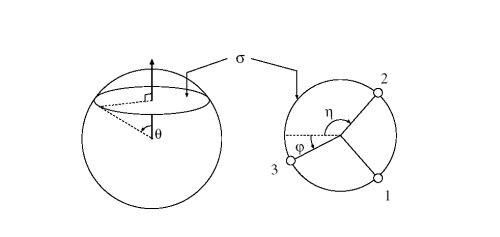Figure 2: Definition of the three angles used to describe the three
electrons on a sphere.The three electrons form a σ plane. On the
plane (the right figure) the three electrons are confined to a circle.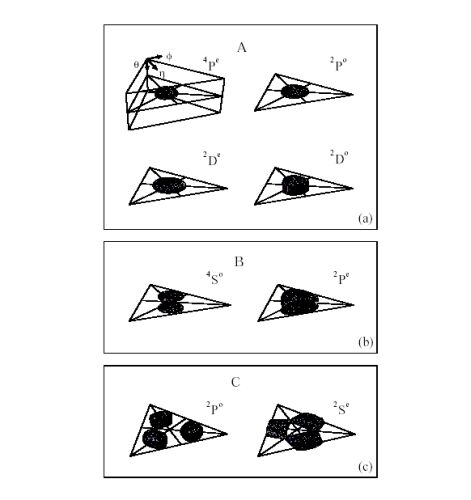Figure 3: The equidensity surface plots of the three-electron wavefunctions for the
eight intrashell states at r1 = r2 = r3. The surface represents 60% of the maximum density.
Each slice represents the whole range of the three angles (0≤θ≤π, 0≤η≤π, -η≤φ≤η).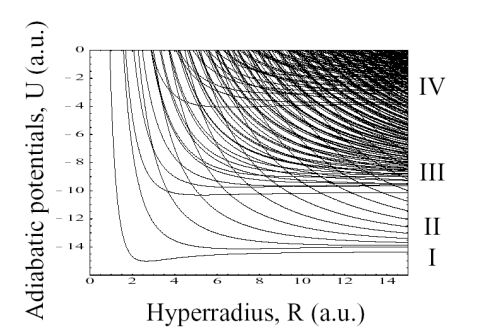Figure 4: Hyperspherical adiabatic potential curves for the 1Se symmetry of Be within the s4 configuration.

References:

 W. Cooper, U. Fano, and F. Prats, Phys. Rev. Lett. 10, 518 (1963).
 U. Fano, Phys. Today 29, 32 (1976).
 J. Macek, J. Phys. B1, 831 (1968).
 T. Morishita and C. D. Lin,Phys. Rev. A, 57, 1835 (1999).

This work was supported by the Chemical Sciences, Geosciences and Biosciences Division,
Office of Basic Energy Sciences, Office of Science, U.S. Department of Energy.

Submitted to the Fano Memorial Symposium, July 2002 in Cambridge, MA.

This abstract is also available in Postscript or Adobe Acrobat formats.

 Return to do another abstract search of all our holdings.